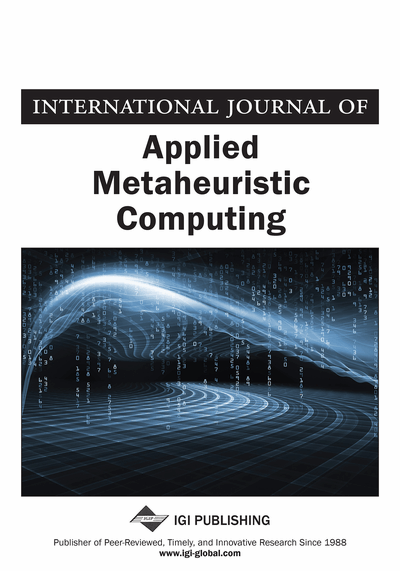DIMMA-Implemented Metaheuristics for Finding Shortest Hamiltonian Path Between Iranian Cities Using Sequential DOE Approach for Parameters Tuning

Masoud Yaghini (Iran University of Science and Technology, Iran), Mohsen Momeni (Iran University of Science and Technology, Iran) and Mohammadreza Sarmadi (Iran University of Science and Technology, Iran)
DOI: 10.4018/jamc.2011040104
Available
\$37.50
No Current Special Offers

Abstract

A Hamiltonian path is a path in an undirected graph, which visits each node exactly once and returns to the starting node. Finding such paths in graphs is the Hamiltonian path problem, which is NP-complete. In this paper, for the first time, a comparative study on metaheuristic algorithms for finding the shortest Hamiltonian path for 1071 Iranian cities is conducted. These are the main cities of Iran based on social-economic characteristics. For solving this problem, four hybrid efficient and effective metaheuristics, consisting of simulated annealing, ant colony optimization, genetic algorithm, and tabu search algorithms, are combined with the local search methods. The algorithms’ parameters are tuned by sequential design of experiments (DOE) approach, and the most appropriate values for the parameters are adjusted. To evaluate the proposed algorithms, the standard problems with different sizes are used. The performance of the proposed algorithms is analyzed by the quality of solution and CPU time measures. The results are compared based on efficiency and effectiveness of the algorithms.
Article Preview
Top

1. Introduction

The shortest Hamiltonian path is a well-known and important combinatorial optimization problem. The goal of Traveling Salesman Problem (TSP) is to find the shortest path that visits each city in a given list exactly once and then returns to the starting city. The distances between n cities are stored in a distance matrix D with elements dij where i, j = 1, 2, ..., n and the diagonal elements dij are zero (Hahsler & Hornik, 2007). In the 1920’s, the mathematician and economist Menger publicized this problem among his colleagues in Vienna. In the 1930’s the problem reappeared in the mathematical circles of Princeton (Applegate et al., 1998). In the 1940’s, mathematician Flood publicized the name, TSP, within the mathematical community at mass (Lawler et al., 1985). Despite this simple problem statement, solving the TSP is difficult, since it belongs to the class of NP-complete problems (Johnson & Papadimitriou, 1985). The importance of the TSP arises besides from its theoretical appeal from the variety of its applications. Typical applications in operations research include vehicle routing, computer wiring, cutting wallpaper, job sequencing, and job scheduling. Recognizing methods to solve TSP and find the appropriate method is one of the important research areas (Hahsler & Hornik, 2009). In this paper, a comparative study is conducted between Simulated Annealing (SA) and Tabu Search (TS) algorithm as single-solution-based algorithms, Ant Colony Optimization (ACO), and Genetic Algorithm (GA) as population-based-algorithms to find the shortest Hamiltonian path for 1071 Iranian cities.

The proposed algorithms are implemented based on Design and Implementation Methodology for Metaheuristic Algorithms (DIMMA). DIMMA has three sequential phases that each of them has several steps (Figure 1). These phases are as follows: initiation, in which the problem in hand must be understood precisely, the goal of designing metaheuristic must be clearly defined, and the instances must be selected. The next phase is blueprint, the important goals of this phase are selecting solution strategy, defining performance measures, and designing algorithm for the solution strategy. The last phase is construction in which implementing the designed algorithm, parameters tuning (parameter setting), analyzing its performance, and finally documentation of results must be done (Yaghini & Kazemzadeh, 2010).

The contribution of our paper could be summarized as follows. In this paper, we proposed four effectiveness and efficient hybrid algorithms for solving TSP problem. High quality of the solution and short computation time show the efficiency and effectiveness of the algorithms. Tuning parameter has been done using DOE based on sequential methodology. Using metaheuristic algorithms with local search to finding the shortest Hamiltonian path between the Iranian cities is the practical contribution of this paper.

The paper is organized as follows. In section two, literature review is conducted. In the third section, the initiation phase of the proposed algorithms is presented. In the fourth section, blueprint, and in the fifth section construction phase of algorithms are discussed. In section six, the shortest Hamiltonian path between Iranian cities is derived by using the proposed algorithms. Conclusion is presented in section seven.

Top

2. Literature Review

The TSP problem has different types, such as Probabilistic Traveling Salesman Problem (PTSP), Traveling Salesman Problem with Time Windows (TSPTW), Multicommodity Traveling Salesman Problem (MTSP), and Railway Traveling Salesman Problem (RTSP).

Complete Article List

Search this Journal:
Reset
Volume 13: 4 Issues (2022): 2 Released, 2 Forthcoming
Volume 12: 4 Issues (2021)
Volume 11: 4 Issues (2020)
Volume 10: 4 Issues (2019)
Volume 9: 4 Issues (2018)
Volume 8: 4 Issues (2017)
Volume 7: 4 Issues (2016)
Volume 6: 4 Issues (2015)
Volume 5: 4 Issues (2014)
Volume 4: 4 Issues (2013)
Volume 3: 4 Issues (2012)
Volume 2: 4 Issues (2011)
Volume 1: 4 Issues (2010)
View Complete Journal Contents Listing